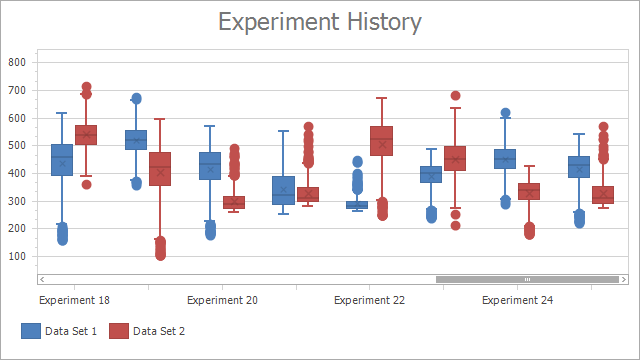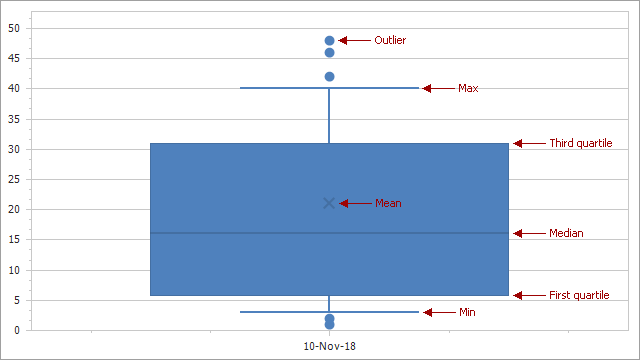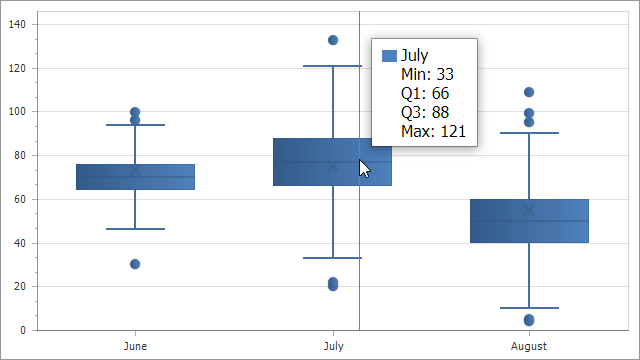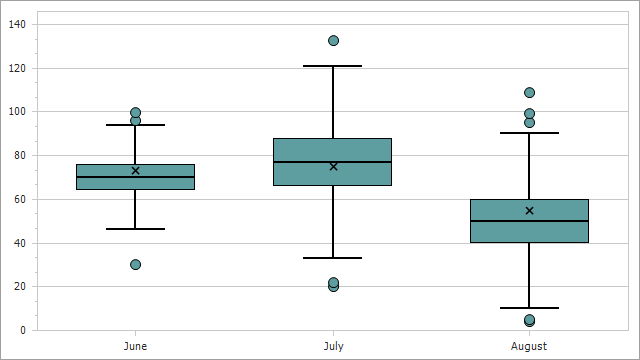## End-User Documentation

20.1 (EAP/Beta)
19.2
19.1
The page you are viewing does not exist in version 19.1. This link will take you to the root page.
18.2
The page you are viewing does not exist in version 18.2. This link will take you to the root page.
18.1
The page you are viewing does not exist in version 18.1. This link will take you to the root page.
17.2
The page you are viewing does not exist in version 17.2. This link will take you to the root page.

# Box Plot Chart

The Box Plot (also called Box-and-Whisker Plot) chart is used to analyze statistic-driven data points. To draw a Box Plot point, you must pass the Min, Quartile1, Median, Quartile3 and Max parameters. You can optionally display a set of Outliers and a Mean value.Demo: Box Plot

## Chart Type Characteristics

The table below lists the main box plot chart characteristics:

Feature Value
Series view type BoxPlotSeriesView
Diagram type 2D XYDiagram
Number of arguments per series point 1
Number of values per series point 7

The following image shows the Box Plot chart elements:## Limitations

The Box Plot series view does not support series labels.

## Create a Box Plot Chart

The following example demonstrates how to create a Box Plot chart with three points:

• Add a chart to the WebForms project and specify the chart's data source.
• Add a series to the chart. Set its view type to BoxPlot.
• Specify data members for the series.

You can call the SeriesBase.SetBoxPlotDataMembersWithOutliers method to pass all the data member names as parameters. To create a chart without outliers, use the SeriesBase.SetBoxPlotDataMembers method.

To keep the Mean parameter undefined, pass the MeanDataMember parameter as null or an empty string to the SeriesBase.SetBoxPlotDataMembersWithOutliers (SeriesBase.SetBoxPlotDataMembers) method. If you manually add SeriesPoint objects to the Points collection, you can set the SeriesPoint.Values value to double.NaN, DateTime.MinValue or TimeSpan.Zero.

``````using DevExpress.XtraCharts;
using DevExpress.XtraCharts.Web;
using System;
using System.Collections.Generic;

namespace BoxPlotChart {
public partial class WebForm1 : System.Web.UI.Page {
protected void Page_Load(object sender, EventArgs e) {
WebChartControl chart = new WebChartControl();
chart.Height = 450;
chart.Width = 800;
Series series = new Series("Box Plot", ViewType.BoxPlot);
series.DataSource = DataPoint.GetDataPoints();
series.SetBoxPlotDataMembersWithOutliers("Argument", "Min", "Quartile1", "Median", "Quartile3", "Max", "Mean", "Outliers");
}
}
public class DataPoint {
public string Argument { get; set; }
public double Min { get; set; }
public double Max { get; set; }
public double Quartile1 { get; set; }
public double Quartile3 { get; set; }
public double Median { get; set; }
public double Mean { get; set; }
public double[] Outliers { get; set; }
public DataPoint(string argument, double min, double max, double q1, double q3, double median, double mean, double[] outliers) {
this.Argument = argument;
this.Min = min;
this.Max = max;
this.Quartile1 = q1;
this.Quartile3 = q3;
this.Median = median;
this.Mean = mean;
this.Outliers = outliers;
}
public static List<DataPoint> GetDataPoints() {
List<DataPoint> data = new List<DataPoint> {
new DataPoint("June", 46, 94, 64, 76, 70, 73, new double[]{ 30, 96.3, 99.56 }),
new DataPoint("July", 33, 121, 66, 88, 77, 75, new double[]{ 20, 22, 132.7 }),
new DataPoint("August", 10, 90, 40, 60, 50, 55, new double[]{ 4, 5, 95.4, 99.3, 109 })
};
return data;
}
}
}
``````

## Format the Crosshair Label

Use the SeriesBase.CrosshairLabelPattern property to format the crosshair label text.The Chart Control provides the following placeholders that you can use in patterns:

Placeholder Description
{BP_MIN} Displays the Box Plot point's Minimum value.
{BP_Q1} Displays the Box Plot point's First Quartile value.
{BP_MDN} Displays the Box Plot point's Median value.
{BP_AVG} Displays the Box Plot point's Mean value.
{BP_Q3} Displays the Box Plot point's Third Quartile value.
{BP_MAX} Displays the Box Plot point's Maximum value.
``````series.CrosshairLabelPattern = "{A}" + Environment.NewLine +
"Min: {BP_MIN}" + Environment.NewLine +
"Q1: {BP_Q1}" + Environment.NewLine +
"Q3: {BP_Q3}" + Environment.NewLine +
"Max: {BP_MAX}";
``````

You can use format specifiers to format values that the placeholders show. For example, the "{BP_Q1:f1}" pattern displays the First Quartile value with one digit after a comma.

## Change Chart Appearance

This section explains how to modify the Box Plot chart appearance as follows:The BoxPlotSeriesView class exposes the following appearance settings for the Box Plot chart:

``````BoxPlotSeriesView view = series.View as BoxPlotSeriesView;